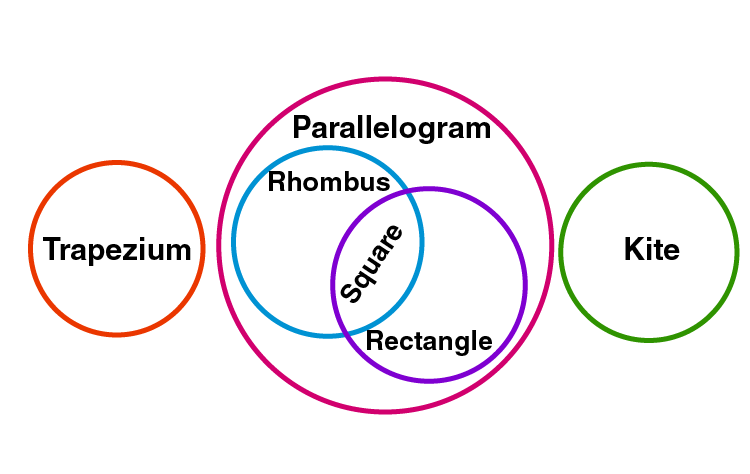# Quadrilaterals Class 9 Notes - Chapter 8

## CBSE Class 9 Maths Quadrilaterals Notes:-

Get the complete notes on quadrilaterals class 9 here. A quadrilateral is a shape which has four sides. In this article, we are going to discuss the different types of quadrilaterals such as square, rectangle, parallelogram properties with proofs.

To know more about Parallelogram, visit here.

### Parallelogram: Opposite sides of a parallelogram are equal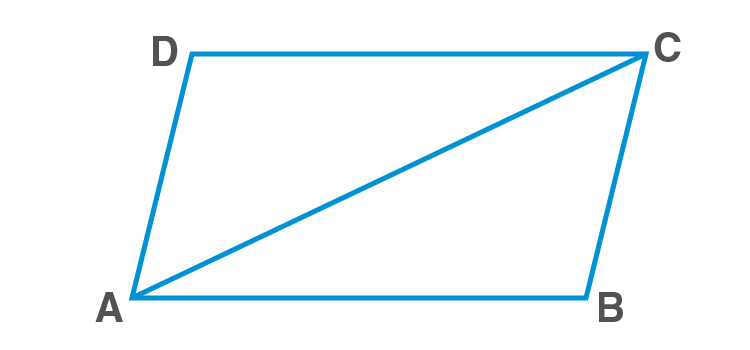In ΔABC and ΔCDA

AC=AC [Common / transversal]

BCA=DAC [alternate angles]

BAC=DCA [alternate angles]

ΔABCΔCDA  [ASA rule]

Hence,

### Opposite angles in a parallelogram are equal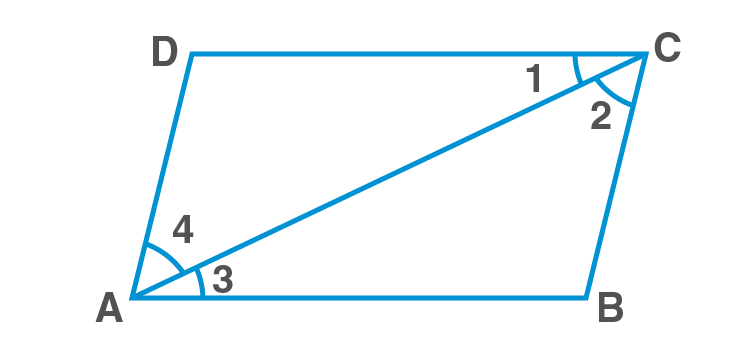In parallelogram ABCD

ABCD; and AC is the transversal

Hence, 1=3….(1) (alternate interior angles)

BCDA; and AC is the transversal

Hence, 2=4….(2) (alternate interior angles)

1+2=3+4

Similarly,

### Properties of diagonal of a parallelogram

Diagonals of a parallelogram bisect each other.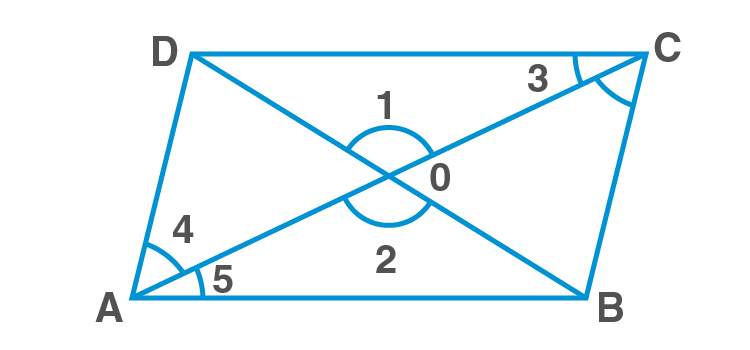In  ΔAOB and ΔCOD,

3=5 [alternate interior angles]

1=2  [vertically opposite angles]

AB=CD [opp. Sides of parallelogram]

ΔAOBΔCOD [AAS rule]

OB=OD and OA=OC [C.P.C.T]

Hence, proved

Conversely,
If the diagonals of a quadrilateral bisect each other, then it is a parallelogram.

Diagonal of a parallelogram divides it into two congruent triangles.In ΔABC and ΔCDA,

AB=CD [Opposite sides of parallelogram]

AC=AC [Common side]

ΔABCΔCDA [by SSS rule]

Hence, proved.

#### For more information on Properties Of Parallelogram, watch the below video### Diagonals of a rhombus bisect each other at right angles

Diagonals of a rhombus bisect each – other at right angles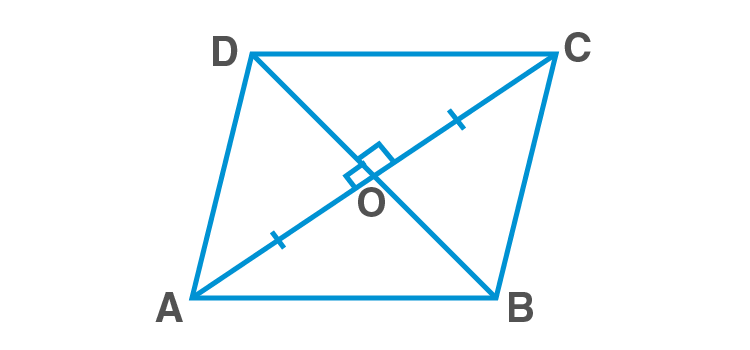In ΔAOD and ΔCOD,

OA=OC [Diagonals of parallelogram bisect each other]

OD=OD [Common side]

ΔAODΔCOD [SSS rule]

AOD=DOC [C.P.C.T]

AOD+DOC=180 [ AOC is a straight line]

Hence, AOD=DOC=90

Hence proved.

#### For more information on Properties Of Rhombus, watch the below video### Diagonals of a rectangle bisect each other and are equal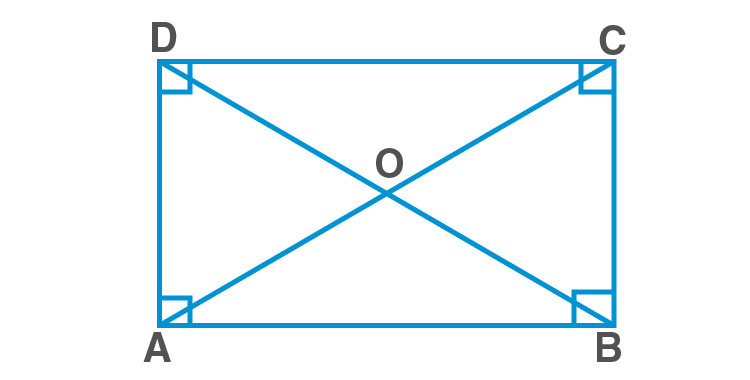Rectangle ABCD

AB=BA [Common side]

BC=AD [Opposite sides of a rectangle]

ABC=BAD [Each = 900  ABCD is a Rectangle]

AC=BD  [C.P.C.T]

AD=CB  [Opposite sides of a rectangle]

ODA=OBC  [ AD||BC and transversal BD intersects them]

OA=OC  [C.P.C.T]

Similarly we can prove OB=OD

#### For more information on Properties Of Rectangle, watch the below video### Diagonals of a square bisect each other at right angles and are equal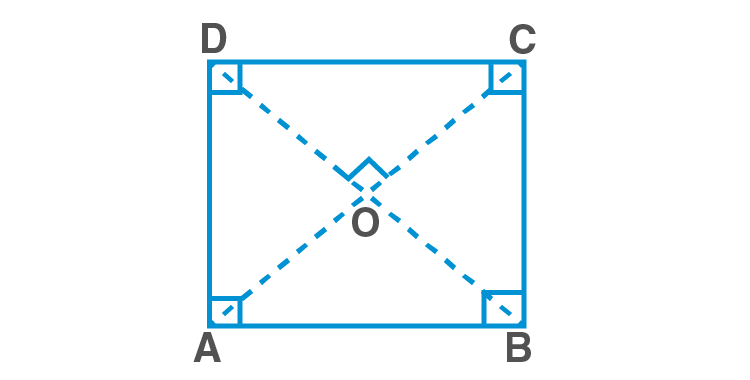Square ABCD

AB=BA [Common side]

BC=AD [Opposite sides of a Square]

ABC=BAD [Each = 900  ABCD is a Square]

AC=BD  [C.P.C.T]

AD=CB  [Opposite sides of a Square]

ODA=OBC  [ AD||BC and transversal BD intersects them]

OA=OC  [C.P.C.T]

Similarly we can prove OB=OD

In ΔOBA and ΔODA,

OB=OD   [ proved above]

BA=DA  [Sides of a Square]

OA=OA  [ Common side]

ΔOBAΔODA,  [ SSS rule]

AOB=AOD  [ C.P.C.T]

But, AOB+AOD=1800  [ Linear pair]

AOB=AOD=900

### Important results related to parallelograms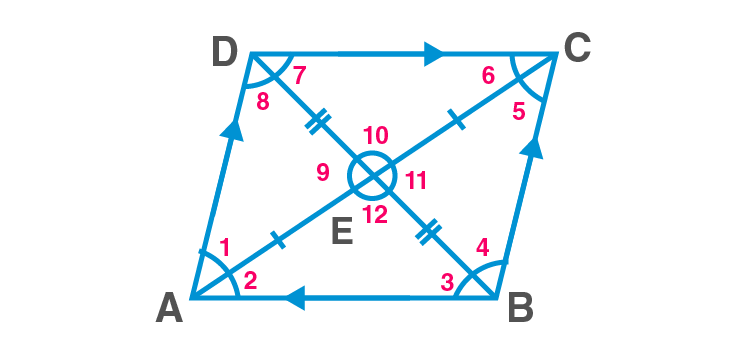Parallelogram ABCD

Opposite sides of a parallelogram are parallel and equal.

Opposite angles of a parallelogram are equal adjacent angels are supplementary.

A=C,B=D,

A+B=1800,B+C=1800,C+D=1800,D+A=1800

A diagonal of parallelogram divides it into two congruent triangles.

ΔABCΔCDA [With respect to AC as diagonal]

ΔADBΔCBD   [With respect to BD as diagonal]

The diagonals of a parallelogram bisect each other.

AE=CE,BE=DE

1=5 (alternate interior angles)

2=6 (alternate interior angles)

3=7 (alternate interior angles)

4=8 (alternate interior angles)

9=11 (vertically opp. angles)

10=12 (vertically opp. angles)

## The Mid-Point Theorem

The line segment joining the midpoints of two sides of a triangle is parallel to the third side and is half of the third side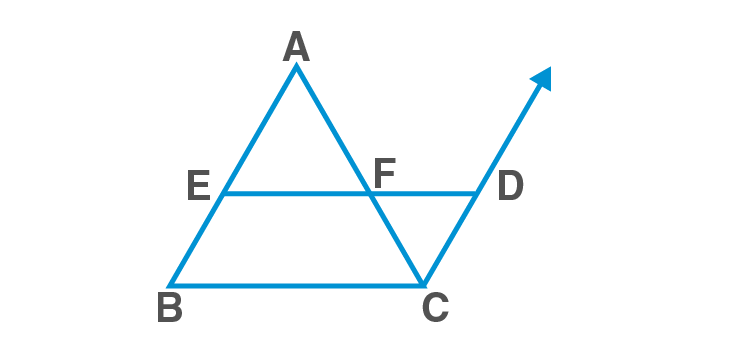In ΔABCE – the midpoint of ABF – the midpoint of AC

Construction: Produce EF to D such that EF=DF.

In ΔAEF and ΔCDF,

AF=CF  [ F is the midpoint of AC]

AFE=CFD  [ V.O.A]

EF=DF [ Construction]

ΔAEFΔCDF [SAS rule]

Hence,

EAF=DCF….(1)

DC=EA=EB  [ E is the midpoint of AB]

DCEAAB [Since, (1), alternate interior angles]

DCEB

So EBCD is a parallelogram

Therefore, BC=ED and BCED

Since, ED=EF+FD=2EF=BC  [ EF=FD]

We have,EF=12BC and EF||BC

Hence proved.

To know more about Mid-Point Theorem, visit here.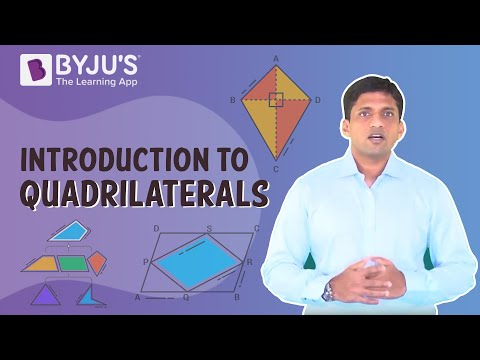Any four points in a plane, of which three are non-collinear are joined in order results into a four-sided closed figure called ‘quadrilateral’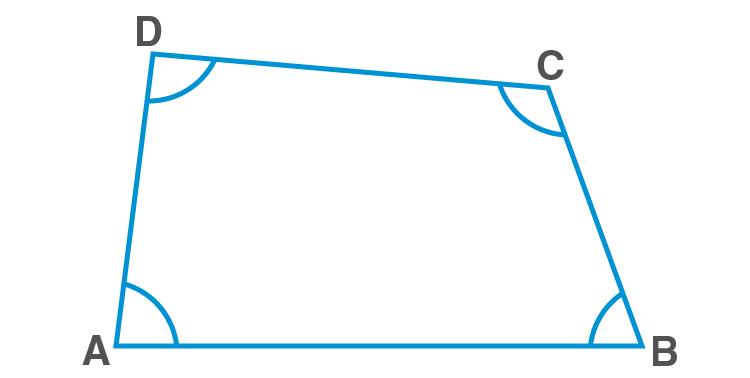### Angle sum property of a quadrilateral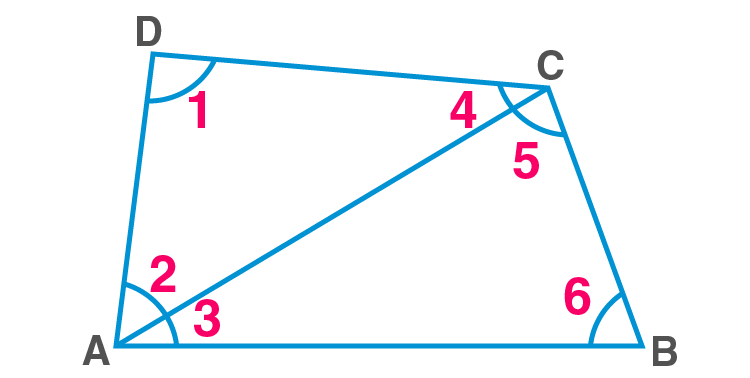Angle sum property – Sum of angles in a quadrilateral is 360

1+2+4=180 (Angle sum property of triangle)…………….(1)

In ABC,

3+5+6=180 (Angle sum property of triangle)………………(2)

(1) + (2):

1+2+3+4+5+6=360

I.e, A+B+C+D=360

Hence proved

### Trapezium

A trapezium is a quadrilateral with any one pair of opposite sides parallel.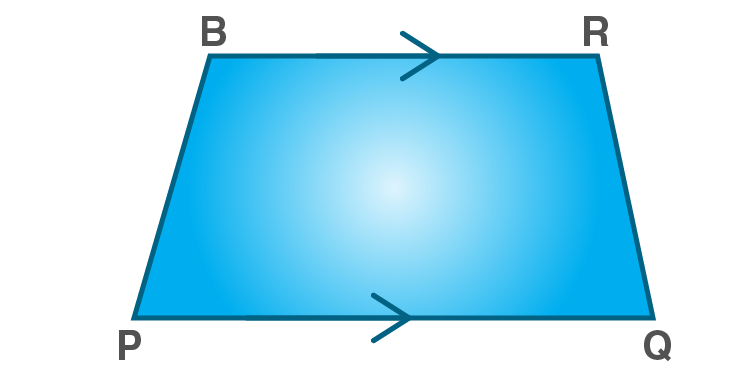Trapezium

PQRS is a trapezium in which PQ||RS

### Parallelogram

A parallelogram is a quadrilateral, with both pair of opposite sides parallel and equal. In a parallelogram, diagonals bisect each other.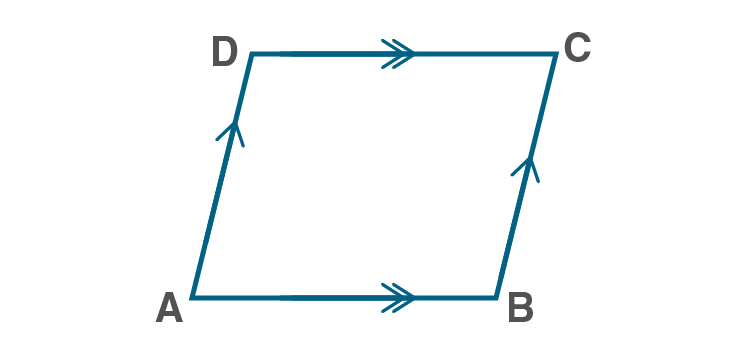Parallelogram ABCD

### Rhombus

A rhombus is a parallelogram with all sides equal. In a rhombus, diagonals bisect each other perpendicularly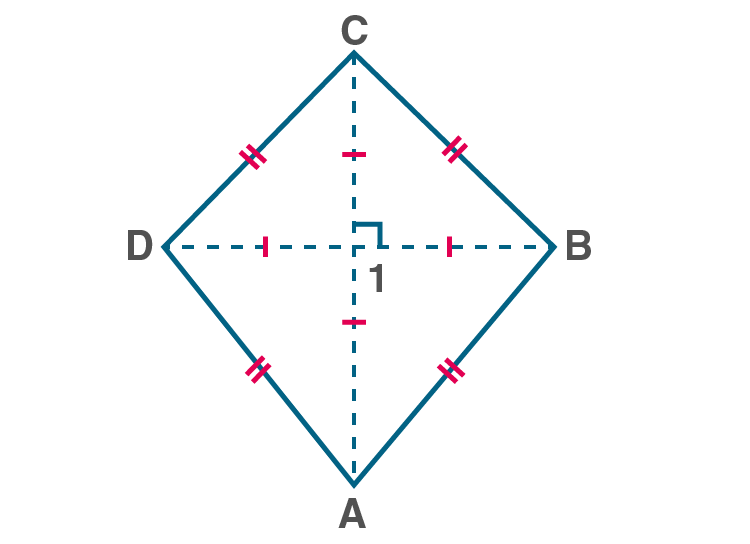Rhombus ABCD

A rhombus ABCD in which AB=BC=CD=AD and  ACBD

### Rectangle

A rectangle is a parallelogram with all angles as right angles.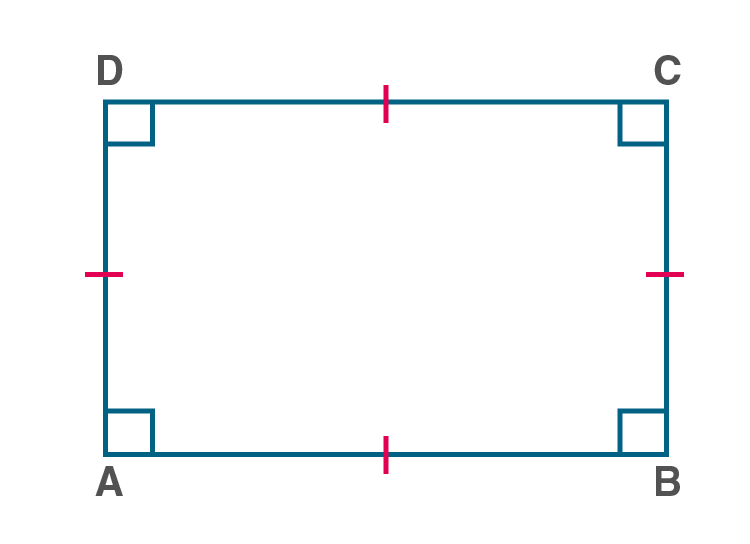Rectangle ABCD

A rectangle ABCD in which, A=B=C=D=900

### Square

A square is a special case of a parallelogram with all angles as right angles and all sides equal.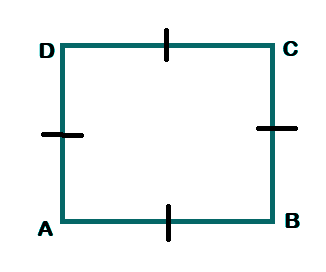Square ABCD

A square ABCD in which A=B=C=D=900 and AB=BC=CD=AD

### Kite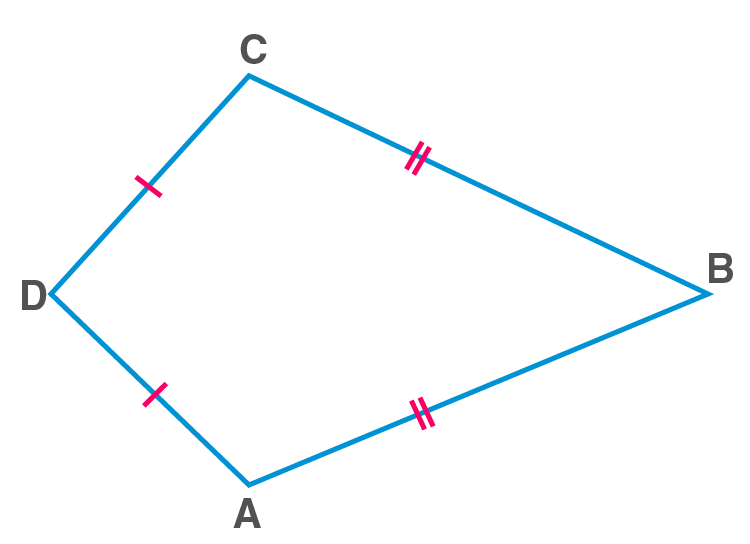Kite ABCD

A kite ABCD in which AB=BC and AD=CD